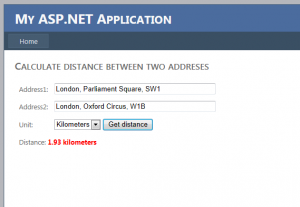Lets start with building the empty asp.net website. After creating basic UI controls we can implement the back end of our application. We will use Json serialization when getting response from API so we need to prepare serialization function and geolocation response class.

``` public static T FromJSON<T>(string json)
{
JavaScriptSerializer jss = new JavaScriptSerializer();

return jss.Deserialize<T>(json);
}
```

We will use data contracts to deserialize Json response to our class

``` [DataContract(Namespace = "")]
public class GeocodeResponse
{
[DataMember(Name = "status", Order = 1)]
public string Status { get; set; }

[DataMember(Name = "result", Order = 2)]
public List<Result> Results { get; set; }

[DataContract(Name = "result", Namespace = "")]
public class Result
{
[DataMember(Name = "geometry")]
public CGeometry Geometry { get; set; }

[DataContract(Name = "geometry", Namespace = "")]
public class CGeometry
{
[DataMember(Name = "location")]
public CLocation Location { get; set; }

[DataContract(Name = "location", Namespace = "")]
public class CLocation
{
[DataMember(Name = "lat", Order = 1)]
public double Lat { get; set; }
[DataMember(Name = "lng", Order = 2)]
public double Lng { get; set; }
}
}
}
}
```

To get Json object from API we need to send request and read response from it.

``` public static string WebRequestJson(string url)
{
var response = string.Empty;

StreamWriter requestWriter;

var webRequest = System.Net.WebRequest.Create(url) as HttpWebRequest;
if (webRequest != null)
{
webRequest.Method = "POST";
webRequest.ServicePoint.Expect100Continue = false;
webRequest.Timeout = 99000;

webRequest.ContentType = "application/json";
//POST the data.
using (requestWriter = new StreamWriter(webRequest.GetRequestStream()))
{
requestWriter.Write("");
}
}

HttpWebResponse resp = (HttpWebResponse)webRequest.GetResponse();
using (Stream resStream = resp.GetResponseStream())
{
{
}
}

return response;
}
```

In our main function we are getting Json objects from API by making request to Google and passing address in request URL. Having Json objects for each address we deserialize it to our GeocodeResponse class. In this step we can check response if it is valid or contains only one location for one provided address.

``` public static double GetDistance(string address1, string address2, DistanceUnit unit)
{
//make jason requests to google api
string json1 = WebRequestJson(
);
string json2 = WebRequestJson(
);

//deserialize json to GeocodeResponse object
var loc1 = FromJSON<GeocodeResponse>(json1);
var loc2 = FromJSON<GeocodeResponse>(json2);

//check is response is ok
if (loc1.Status != "OK" || loc2.Status != "OK") { return -1; }

//check if only one location found for each address
//if (loc1.Results.Count > 1 || loc2.Results.Count > 1) { return -2; }

//copy coordinate values
var pos1 = new Coordinate()
{
Latitude = loc1.Results.First().Geometry.Location.Lat,
Longitude = loc1.Results.First().Geometry.Location.Lng
};
var pos2 = new Coordinate()
{
Latitude = loc2.Results.First().Geometry.Location.Lat,
Longitude = loc2.Results.First().Geometry.Location.Lng
};

//return distance in unit
return CalculateDistance(pos1, pos2, unit);
}
```

When we have the final coordinates (latitude and longitude) for each address, we can now calculate the distance in the specified unit. We take the earth’s radius into account- the rest it’s just the pure mathematics.

```  public static double CalculateDistance(Coordinate pos1, Coordinate pos2, DistanceUnit unit)
{
var R = 6371;//default in km

switch (unit)
{
case DistanceUnit.Miles:
R = 3960; break;
case DistanceUnit.Kilometers:
R = 6371; break;
case DistanceUnit.Meters:
R = 6371000; break;
}

//get location difference and convert to radians
var dLat = DegreeToRadian(pos2.Latitude - pos1.Latitude);
var dLon = DegreeToRadian(pos2.Longitude - pos1.Longitude);

//calculate distance
var a = Math.Sin(dLat / 2) * Math.Sin(dLat / 2) +
Math.Sin(dLon / 2) * Math.Sin(dLon / 2);

var c = 2 * Math.Asin(Math.Min(1, Math.Sqrt(a)));

var d = R * c;//convert to unit

return d;
}
```

When we have all implementation done, we can finally test our application. I have included complete working application for your tests.

Geolocation(No Ratings Yet)Loading...
Tags: